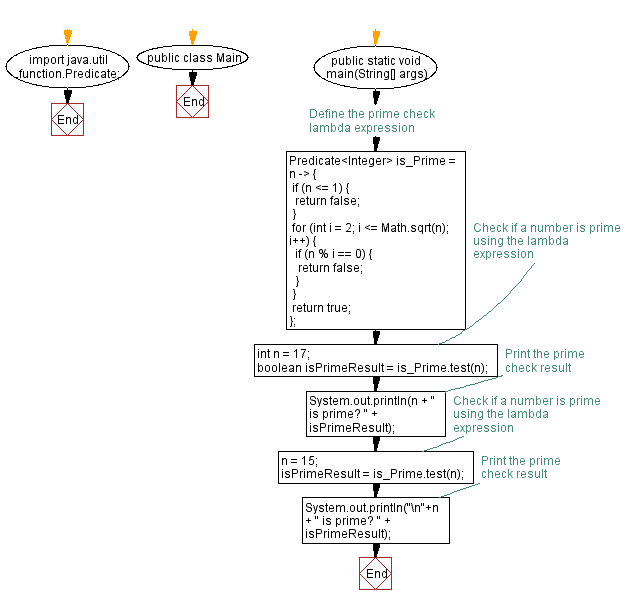# Check prime number using Lambda expression in Java

## Java Lambda Program: Exercise-9 with Solution

Write a Java program to implement a lambda expression to create a lambda expression to check if a number is prime.

Sample Solution:

Java Code:

``````import java.util.function.Predicate;

public class Main {
public static void main(String[] args) {
// Define the prime check lambda expression
Predicate<Integer> is_Prime = n -> {
if (n <= 1) {
return false;
}
for (int i = 2; i <= Math.sqrt(n); i++) {
if (n % i == 0) {
return false;
}
}
return true;
};

// Check if a number is prime using the lambda expression
int n = 17;
boolean isPrimeResult = is_Prime.test(n);
// Print the prime check result
System.out.println(n + " is prime? " + isPrimeResult);
// Check if a number is prime using the lambda expression
n = 15;
isPrimeResult = is_Prime.test(n);
// Print the prime check result
System.out.println("\n"+n + " is prime? " + isPrimeResult);
}
}
``````

Sample Output:

```17 is prime? true

15 is prime? false
```

Explanation:

In the main method, we define a lambda expression using the Predicate<Integer>. This functional interface represents a predicate (boolean-valued function) of one argument.

The lambda expression checks if a given number n is prime. It first checks if the number is less than or equal to 1, returning false. Then, it uses a for loop to iterate from 2 to the square root of n. It checks if n is divisible by any number within this range. If it is, it gives false, indicating that the number is not prime. Otherwise, it returns true, indicating the number is prime.

After defining the lambda expression, we use it to check if a number is prime. We do this by calling the test method on the lambda expression and passing the number as an argument. The result is stored in the isPrimeResult variable, which is a boolean.

Flowchart:Live Demo:

Java Code Editor:

Improve this sample solution and post your code through Disqus

Java Lambda Exercises Previous: Calculate factorial using Lambda expression in Java.
Java Lambda Exercises Next: Concatenate strings using Lambda expression in Java.

What is the difficulty level of this exercise?

Test your Programming skills with w3resource's quiz.

﻿

## Java: Tips of the Day

How do I remove repeated elements from ArrayList?

If you don't want duplicates in a Collection, you should consider why you're using a Collection that allows duplicates. The easiest way to remove repeated elements is to add the contents to a Set (which will not allow duplicates) and then add the Set back to the ArrayList:

```Set<String> set = new HashSet<>(yourList);
yourList.clear();